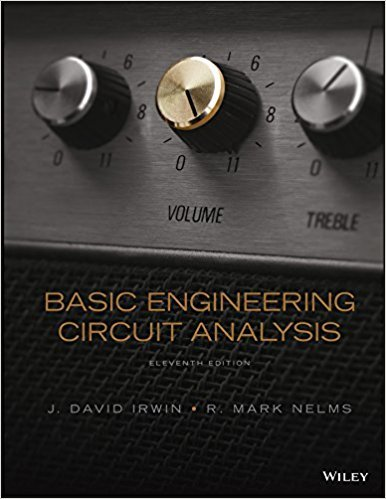×
Get Full Access to Basic Engineering Circuit Analysis - 11 Edition - Chapter 3 - Problem 3.15
Get Full Access to Basic Engineering Circuit Analysis - 11 Edition - Chapter 3 - Problem 3.15

×

# Find Io in the network in Fig. P3.15 using nodal analysis.ISBN: 9781118539293 159

## Solution for problem 3.15 Chapter 3

Basic Engineering Circuit Analysis | 11th Edition

• Textbook Solutions
• 2901 Step-by-step solutions solved by professors and subject experts
• Get 24/7 help from StudySoup virtual teaching assistantsBasic Engineering Circuit Analysis | 11th Edition

4 5 1 368 Reviews
15
2
Problem 3.15

Find Io in the network in Fig. P3.15 using nodal analysis. 1 k 1 k 2 k 4 mA 6 V 2 mA Io + Figure P3.15

Step-by-Step Solution:
Step 1 of 3

4. Find the equivalent resistance R ab for these circuits: Solution: 5. Use the node voltage method to find the power developed in the 20V source. Is there a supernode in this circuit If so, identify it. There is a supernode and a quasi-supernode present in the circuit. The supernode is shown surrounded by a dotted line. As a result of these, There are only two independent nodes (in addition to the reference node) in the circuit….so there will be only two node equations:

Step 2 of 3

Step 3 of 3

##### ISBN: 9781118539293

Unlock Textbook Solution